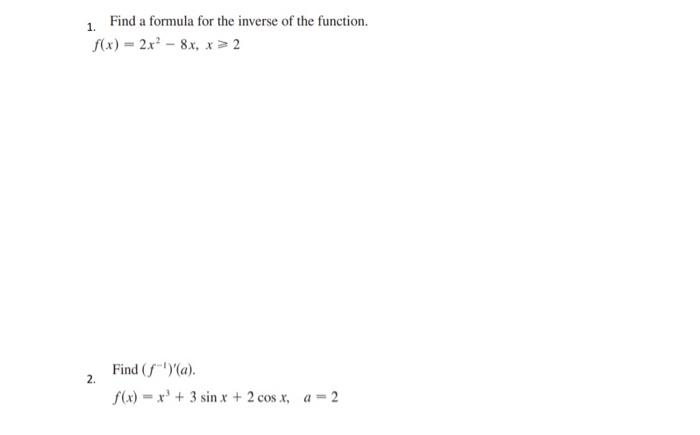### Create an Account

Already have account?

### Forgot Your Password ?

Home / Questions / 1. 1. Find a formula for the inverse of the function. f(x) = 2x² - 8x, x > 2 2. Find ()a)....

# 1. 1. Find a formula for the inverse of the function. f(x) = 2x² - 8x, x > 2 2. Find ()a). f(x) = x + 3 sin x + 2 cos X, a = 2

1. 1. Find a formula for the inverse of the function. f(x) = 2x² - 8x, x > 2 2. Find ()a). f(x) = x + 3 sin x + 2 cos X, a = 2Apr 13 2021 View more View Less

#### Answer (Solved)Subscribe To Get Solution